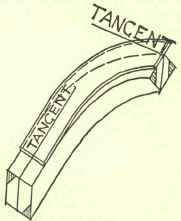Fig. 172.

To obtain the width of the mould at the end a", produce the tangent a"b", and from c" draw c"H perpendicular to it. Take the distance c"H with a pair of compasses, and from any point L in the line B"B" (3), and with radius c"H, strike an arc cutting the line A"A" in M. Join LM, and with any point in LM as centre describe a circle with its diameter equal to the width of the rail on plan. Draw lines tangentially to this circle and parallel to LM, cutting the line A"A" at N and P. Then with NM or NP as radius describe a circle with its centre on b"a" produced, as shown at (4), Fig. 172, and draw lines tangentially to this circle and parallel to a"b", cutting the line Oa" at Q and R. Then QR is the width of the mould on the springing line.

Similar construction is shown for finding the points S and T at the other end of the face mould. If lines drawn through Q and R parallel to a"c" pass through the points S and T respectively the accuracy of the setting-out will be proved. The points Q, R, S, and T are the points from which the elliptical curves are struck. To strike the curve RT, produce RO to U, making OU equal to RO, and draw RV and TV respectively parallel to OT and OR. Divide OT into any convenient number of equal parts, and TV into the same number of equal parts. From R draw lines to the divisions of TV, and from U draw lines through the divisions of OT; the points where these lines cut the first set of lines mark points on the ellipse as shown. The upper curve is set out in a similar manner.

These curves may be checked by finding the axes of the ellipse of which they form part, and trammelling the curves. This is done in the following manner:-Produce cb (3) to meet the level line through a at w. Produce c"b" (4) to w, making b"w equal to bw on the development of the tangents. Join wa", and through O draw Oo parallel to a"w; then Oo is half the minor axis. Mark off the length Oo from the unlettered marked point to its end R on a slip of wood called a "trammel," and place this in the position shown; its length Rr being equal to the length of half the major axis, by placing the trammel in various positions with the two marked points on the axes, the end of the trammel with mark points on the curve of the required ellipse.

The joints are then drawn square with the tangents, and at the same distance from a" and c" as they are from the corresponding points on the development of the tangent. The bevels for setting the ends of the wreath piece are found when finding the width of the end of the face mould. These are shown blacked in at (3).Fig. 173.

Having now obtained our face, mould, and bevels, theory ends and practice begins. The method of using the mould is shown in Fig. 173, the bevels being marked across the end of wreath piece, the shaded portions are worked off, the other angles being "squared" from these. When this has been done the wreath is complete up to the point known as "in the square." Each end should then in turn be jointed temporarily to the straight rail, and the moulding worked a little way on. It is then taken off and the mould completed.